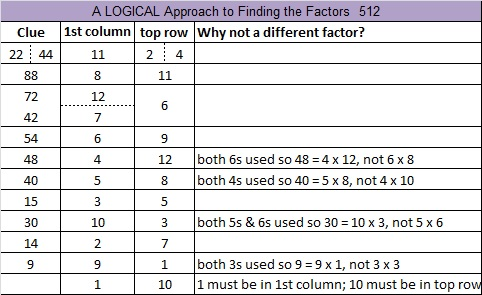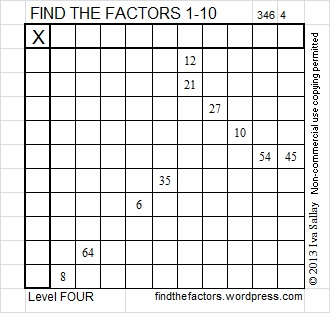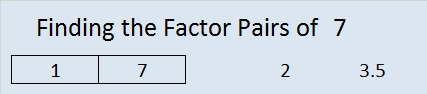# 512 Ants and Level 4The 512 Ants on Sullivan Street is an entertaining book for children that also includes learning activities by Marilyn Burns. The book teaches the important concept of doubling in a very natural way, but it can teach even more than that.  Here is a place value lesson for third grade students that uses the same book. There are relationships between adding, subtracting, doubling, multiplying, dividing, powers, and place value. The book and the place value lesson will make some of those relationships clear.

512 is a perfect cube because 512 = 8^3. It is also a cube of a cube because 8 = 2^3. Thus 512 = (2^3)^3 = 2^9. All of the factors of 512 are powers of 2.Print the puzzles or type the solution on this excel file: 12 Factors 2015-06-01

—————————————————————————————————

• 512 is a composite number.
• Prime factorization: 512 = 2 x 2 x 2 x 2 x 2 x 2 x 2 x 2 x 2, which can be written 512 = (2^9)
• The exponent in the prime factorization is 9. Adding one we get (9 + 1) = 10. Therefore 512 has exactly 10 factors.
• Factors of 512: 1, 2, 4, 8, 16, 32, 64, 128, 256, 512
• Factor pairs: 512 = 1 x 512, 2 x 256, 4 x 128, 8 x 64, or 16 x 32
• Taking the factor pair with the largest square number factor, we get √512 = (√256)(√2) = 16√2 ≈ 22.627417—————————————————————————————————# 7 Spaghetti and Meatballs for All!

### A Wonderful Math-Related Picture Book

Spaghetti and Meatballs for All! by Marilyn Burns is a delightful story, the kind that children enjoy hearing over and over again.

I work at a Leader in Me school, where we promote the Seven Habits. I used this book when I taught about habit 4, think win-win. When we think win-win, we do not allow someone to “step on us’ to give them a win. Mrs. Comfort’s relatives stepped on her over and over again, and they didn’t even realize it. Finally, she cried, “I give up!” and planted herself on a chair. She definitely felt like she was losing. The class listened to the story intently trying to identify places where the Seven Habits were used or could have been used. We had a great discussion afterward. Also since the book did not use the words, “area” or “perimeter” at all, the class hardly realized that the story was also about those concepts. When we followed the suggestions at the back of the book, the class was able to learn about perimeter and area as we had a great discussion about those topics as well.

### Today’s Puzzle:### Factors of 7:

• 7 is a prime number.
• Prime factorization: 7 is prime.
• The exponent of prime number 7 is 1. Adding 1 to that exponent we get (1 + 1) = 2. Therefore 7 has exactly 2 factors.
• Factors of 7: 1, 7
• Factor pairs: 7 = 1 x 7
• 7 has no square factors that allow its square root to be simplified. √7 ≈ 2.64575.How do we know that 7 is a prime number? If 7 were not a prime number, then it would be divisible by at least one prime number less than or equal to √7 ≈ 2.6. Since 7 cannot be divided evenly by 2, we know that 7 is a prime number.

### More about the Number 7:

When 7 is a clue in the FIND THE FACTORS puzzles, one factor will be 7 and the other will be 1.